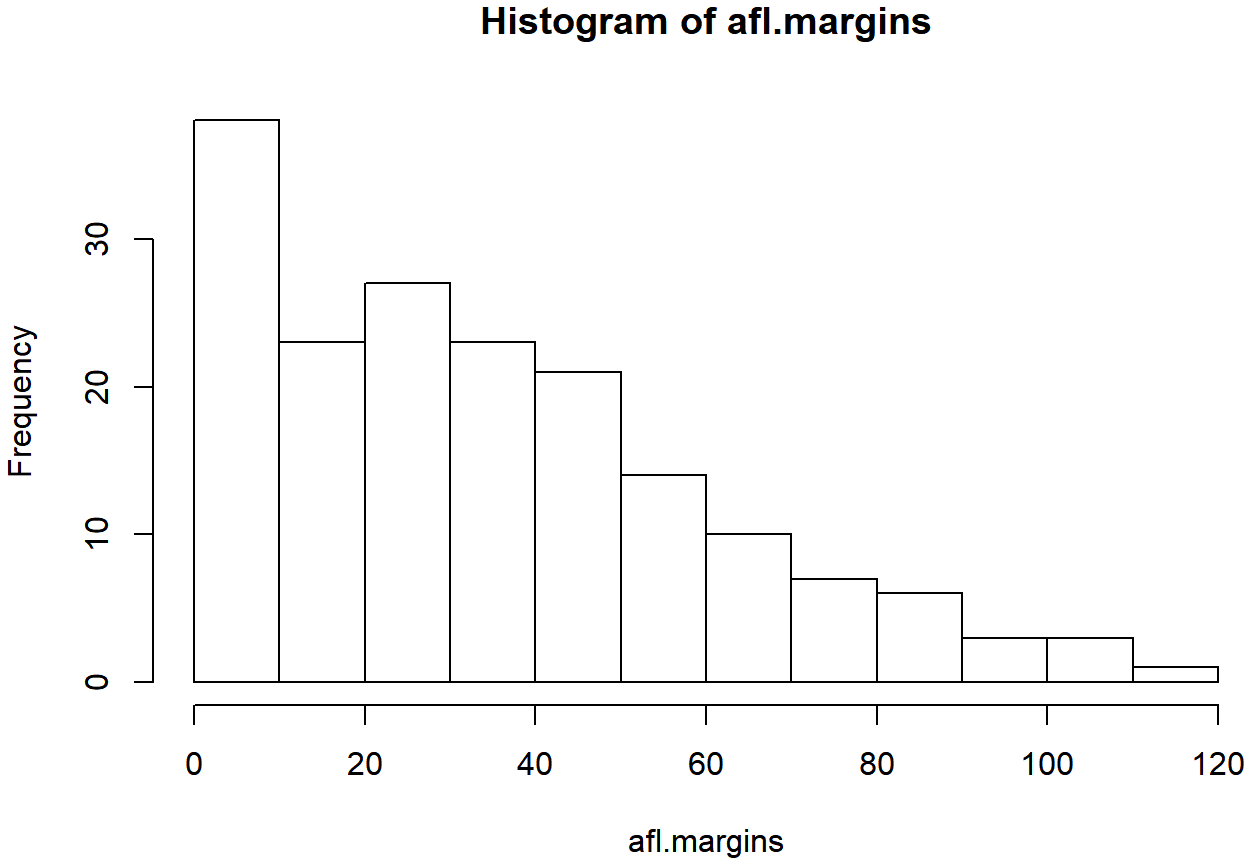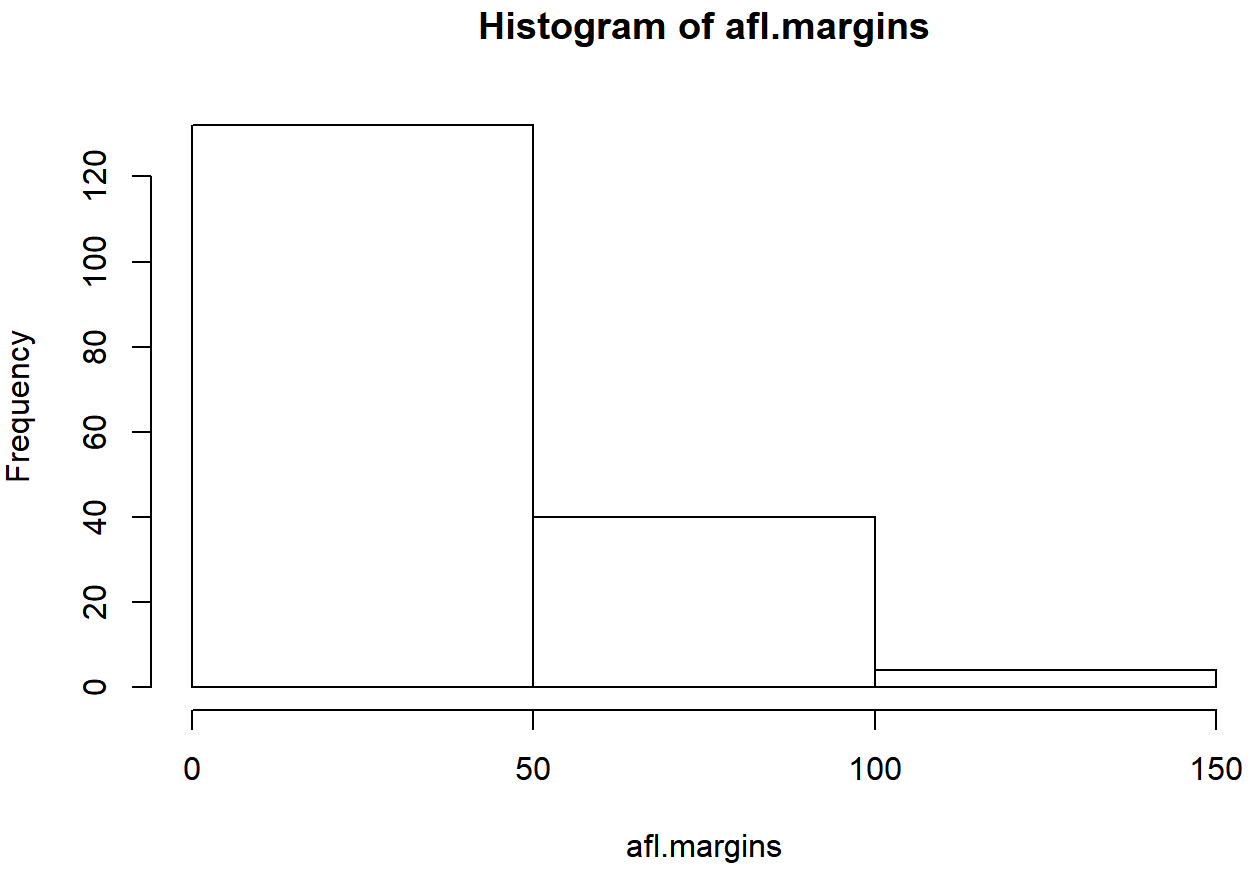# 6.3: Histograms

Now that we’ve tamed (or possibly fled from) the beast that is R graphical parameters, let’s talk more seriously about some real life graphics that you’ll want to draw. We begin with the humble histogram. Histograms are one of the simplest and most useful ways of visualising data. They make most sense when you have an interval or ratio scale (e.g., the afl.margins data from Chapter 5 and what you want to do is get an overall impression of the data. Most of you probably know how histograms work, since they’re so widely used, but for the sake of completeness I’ll describe them. All you do is divide up the possible values into bins, and then count the number of observations that fall within each bin. This count is referred to as the frequency of the bin, and is displayed as a bar: in the AFL winning margins data, there are 33 games in which the winning margin was less than 10 points, and it is this fact that is represented by the height of the leftmost bar in Figure 6.10. Drawing this histogram in R is pretty straightforward. The function you need to use is called hist(), and it has pretty reasonable default settings. In fact, Figure 6.10 is exactly what you get if you just type this:

> hist( afl.margins )   # panel a
load("./rbook-master/data/aflsmall.Rdata")
hist(afl.margins)   # panel aFigure 6.10: The default histogram that R produces

Although this image would need a lot of cleaning up in order to make a good presentation graphic (i.e., one you’d include in a report), it nevertheless does a pretty good job of describing the data. In fact, the big strength of a histogram is that (properly used) it does show the entire spread of the data, so you can get a pretty good sense about what it looks like. The downside to histograms is that they aren’t very compact: unlike some of the other plots I’ll talk about it’s hard to cram 20-30 histograms into a single image without overwhelming the viewer. And of course, if your data are nominal scale (e.g., the afl.finalists data) then histograms are useless.

The main subtlety that you need to be aware of when drawing histograms is determining where the breaks that separate bins should be located, and (relatedly) how many breaks there should be. In Figure 6.10, you can see that R has made pretty sensible choices all by itself: the breaks are located at 0, 10, 20, … 120, which is exactly what I would have done had I been forced to make a choice myself. On the other hand, consider the two histograms in Figure 6.11 and 6.12, which I produced using the following two commands:

hist( x = afl.margins, breaks = 3 )      # panel bFigure 6.11: A histogram with too few bins

hist( x = afl.margins, breaks = 0:116 )  # panel cFigure 6.12: A histogram with too many bins

In Figure 6.12, the bins are only 1 point wide. As a result, although the plot is very informative (it displays the entire data set with no loss of information at all!) the plot is very hard to interpret, and feels quite cluttered. On the other hand, the plot in Figure 6.11 has a bin width of 50 points, and has the opposite problem: it’s very easy to “read” this plot, but it doesn’t convey a lot of information. One gets the sense that this histogram is hiding too much. In short, the way in which you specify the breaks has a big effect on what the histogram looks like, so it’s important to make sure you choose the breaks sensibly. In general R does a pretty good job of selecting the breaks on its own, since it makes use of some quite clever tricks that statisticians have devised for automatically selecting the right bins for a histogram, but nevertheless it’s usually a good idea to play around with the breaks a bit to see what happens.

There is one fairly important thing to add regarding how the breaks argument works. There are two different ways you can specify the breaks. You can either specify how many breaks you want (which is what I did for panel b when I typed breaks = 3) and let R figure out where they should go, or you can provide a vector that tells R exactly where the breaks should be placed (which is what I did for panel c when I typed breaks = 0:116). The behaviour of the hist() function is slightly different depending on which version you use. If all you do is tell it how many breaks you want, R treats it as a “suggestion” not as a demand. It assumes you want “approximately 3” breaks, but if it doesn’t think that this would look very pretty on screen, it picks a different (but similar) number. It does this for a sensible reason – it tries to make sure that the breaks are located at sensible values (like 10) rather than stupid ones (like 7.224414). And most of the time R is right: usually, when a human researcher says “give me 3 breaks”, he or she really does mean “give me approximately 3 breaks, and don’t put them in stupid places”. However, sometimes R is dead wrong. Sometimes you really do mean “exactly 3 breaks”, and you know precisely where you want them to go. So you need to invoke “real person privilege”, and order R to do what it’s bloody well told. In order to do that, you have to input the full vector that tells R exactly where you want the breaks. If you do that, R will go back to behaving like the nice little obedient calculator that it’s supposed to be.

# 6.3.1 Visual style of your histogram

Okay, so at this point we can draw a basic histogram, and we can alter the number and even the location of the breaks. However, the visual style of the histograms shown in Figure @ref(fig:hist1a; hist1b; hist1c) could stand to be improved. We can fix this by making use of some of the other arguments to the hist() function. Most of the things you might want to try doing have already been covered in Section 6.2, but there’s a few new things:

• Shading linesdensityangle. You can add diagonal lines to shade the bars: the density value is a number indicating how many lines per inch R should draw (the default value of NULL means no lines), and the angle is a number indicating how many degrees from horizontal the lines should be drawn at (default is angle = 45 degrees).
• Specifics regarding colourscolborder. You can also change the colours: in this instance the col parameter sets the colour of the shading (either the shading lines if there are any, or else the colour of the interior of the bars if there are not), and the border argument sets the colour of the edges of the bars.
• Labelling the barslabels. You can also attach labels to each of the bars using the labels argument. The simplest way to do this is to set labels = TRUE, in which case R will add a number just above each bar, that number being the exact number of observations in the bin. Alternatively, you can choose the labels yourself, by inputting a vector of strings, e.g., labels = c("label 1","label 2","etc")

Not surprisingly, this doesn’t exhaust the possibilities. If you type help("hist") or ?hist and have a look at the help documentation for histograms, you’ll see a few more options. A histogram that makes use of the histogram-specific customisations as well as several of the options we discussed in Section ?? is shown in Figure ??. The R command that I used to draw it is this:

hist( x = afl.margins,
main = "2010 AFL margins", # title of the plot
xlab = "Margin",           # set the x-axis label
density = 10,              # draw shading lines: 10 per inch
angle = 40,                # set the angle of the shading lines is 40 degrees
border = "gray20",         # set the colour of the borders of the bars
col = "gray80",            # set the colour of the shading lines
labels = TRUE,             # add frequency labels to each bar
ylim = c(0,40)             # change the scale of the y-axis
)Overall, this is a much nicer histogram than the default ones.